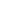New article submisson system
Submit an article in minutesOriginal research

# Prediction of heart disease using forest algorithm over linear regression algorithm using machine learning with improved accuracy

## Abstract

Aim: To perform Predicting heart disease using the Forest algorithm and comparing its feature extraction precision with the Linear Regression Algorithm for improving the accuracy of the prediction. Methods and Materials : In the proposed work, Predicting heart disease was carried out using machine learning algorithms such as Linear Regression (n=10)and Forest algorithm(n=10). Here the pretest power analysis was carried out with 80% and the sample size for the two groups are 20. Results: From The implemented experiment, the Forest algorithm accuracy is 90.32% and the Linear Regression Algorithm 77.21%. There is a statistical 2-tailed significant difference in accuracy for two algorithms is 0.001 (p<0.05) Conclusion:This study concludes that the Forest algorithm on patients healthcare analysis is significantly better than the Linear Regression Algorithm.

## Imprint

K N S Shanmukha Raj, K Thinakaran. Prediction of Heart Disease using Forest Algorithm over Linear Regression Algorithm using Machine Learning With Improved Accuracy. Cardiometry; Issue 25; December 2022; p.1507-1513; DOI: 10.18137/cardiometry.2022.25.15071513; Available from: https://www.cardiometry.net/issues/no25-december-2022/forest-algorithm-linear-regression

## Keywords

Algorithm,   Linear Regression Algorithm,   Predicting Heart Disease,   Machine Learning,   Supervised Classification,   Novel Dimensionality ReductionCurrent issueCardiometry's libraryFounders of Cardiometry